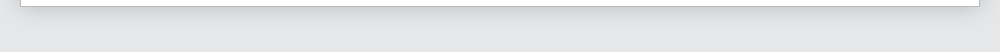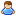Login Products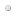SalesSupportDownloadsAbout
 Home » Technical Support » Elevate Web Builder Technical Support » Support Forums » Elevate Web Builder General » View Thread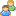View Thread

The following is the text of the current message along with any replies.
 Messages 1 to 2 of 2 total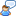Time Diff calculation
 Tue, Feb 27 2018 1:58 PM Permanent Link kamran Hi Trying to calculate the time difference between a start time and an end time for a clock in routine. Here is snippet of my code: -------------------------------------------------------------------------------------------------------------------------------    ClockDataFrm.Clock.Last;    if TimeToStr(ClockDataFrm.Clock.Columns['clock_time_out'].AsDateTime) = '00:00:00' then    begin      ClockDataFrm.Clock.Update;      ClockDataFrm.Clock.Columns['clock_key'].AsString:=ClockKey;      ClockDataFrm.Clock.Columns['clock_time_out'].AsDateTime:=Time();      fails here ---- >ClockDataFrm.Clock.Columns['clock_time_total'].AsDateTime:=ClockDataFrm.Clock.Columns['clock_time_out'].AsDateTime - ClockDataFrm.Clock.Columns['clock_time_in'].AsDateTime;      ClockDataFrm.Clock.Save; ----------------------------------------------------------------------------------------------------------------------------------- it fails at line shown with error :[Error] ClockData.wbs (116,66): Expected date/time or variant but instead found ClockDataFrm.Clock.Columns.Column['clock_time_out'].AsDateTime I would have expected it to calculate the time difference without error as it looks straight forward. the fields clock_time_out, clock_time_in, clock_time_total are all defined in edb as a Type of "Time" in the Clock table thanks Kamran Tue, Feb 27 2018 2:05 PM Permanent Link Tim Young [Elevate Software] Elevate Software, Inc.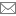timyoung@elevatesoft.com Kamran, << I would have expected it to calculate the time difference without error as it looks straight forward. >> You're trying to assign an Integer (the result of subtracting two DateTime values) to a DateTime property.  You can force the assignment by casting the subtraction to a DateTime value. Tim Young Elevate Software www.elevatesoft.com